# Discussion 3: Querying, Grouping, and Plotting

The problems in this worksheet are taken from past exams. Work on them on paper, since the exams you take in this course will also be on paper.

We encourage you to complete this worksheet in a live discussion section. Solutions will be made available after all discussion sections have concluded. You don’t need to submit your answers anywhere.

Note: We do not plan to cover all problems here in the live discussion section; the problems we don’t cover can be used for extra practice.

## Problem 1

For this question we will use data from the 2021 Women’s National Basketball Association (WNBA) season for the next several problems. In basketball, players score points by shooting the ball into a hoop. The team that scores the most points wins the game.

Kelsey Plum, a WNBA player, attended La Jolla Country Day School, which is adjacent to UCSD’s campus. Her current team is the Las Vegas Aces (three-letter code 'LVA'). In 2021, the Las Vegas Aces played 31 games, and Kelsey Plum played in all 31.

The DataFrame plum contains her stats for all games the Las Vegas Aces played in 2021. The first few rows of plum are shown below (though the full DataFrame has 31 rows, not 5):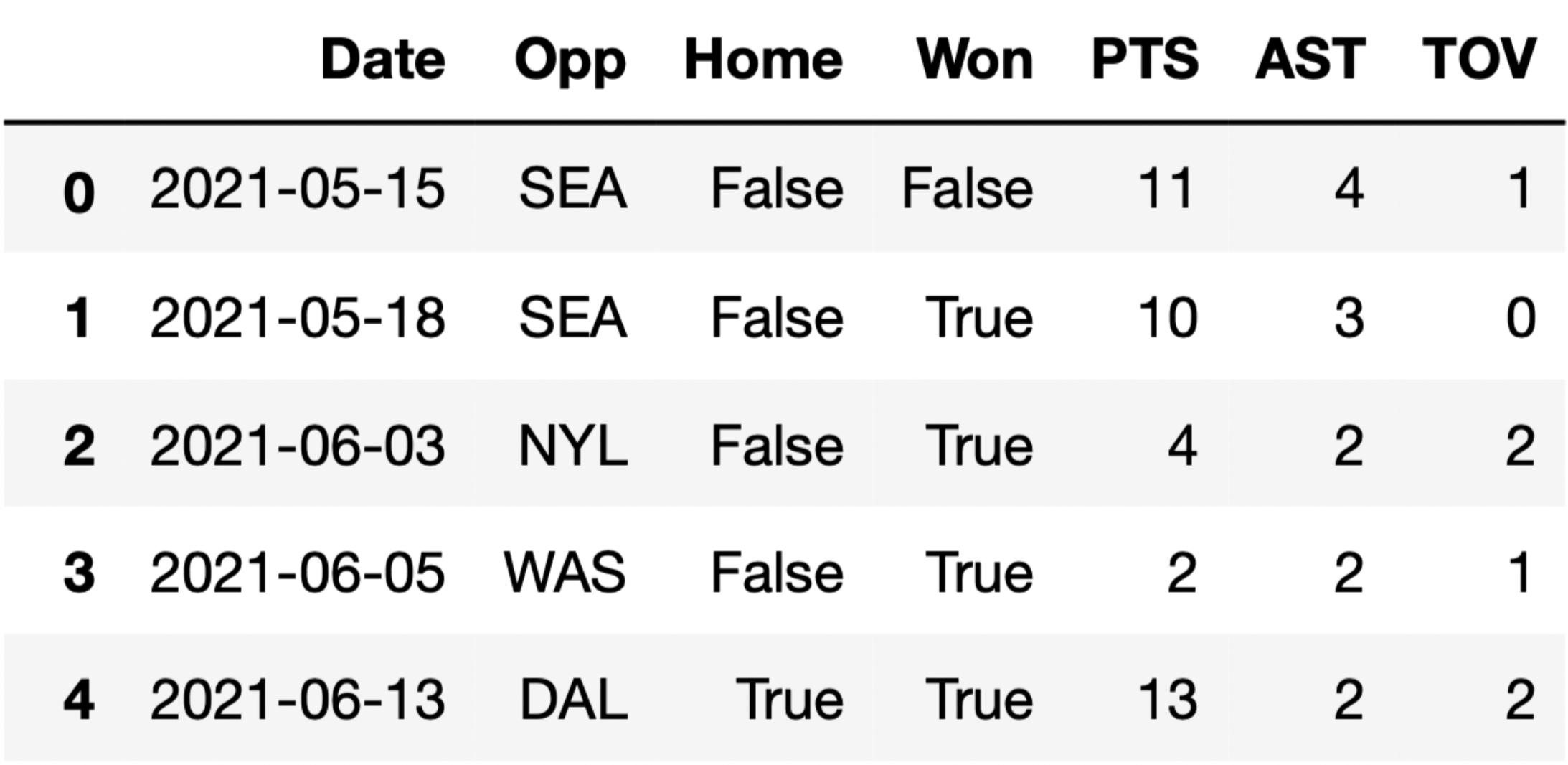Each row in plum corresponds to a single game. For each game, we have:

• 'Date' (str), the date on which the game was played
• 'Opp' (str), the three-letter code of the opponent team
• 'Home' (bool), True if the game was played in Las Vegas (“home”) and False if it was played at the opponent’s arena (“away”)
• 'Won' (bool), True if the Las Vegas Aces won the game and False if they lost
• 'PTS' (int), the number of points Kelsey Plum scored in the game
• 'AST' (int), the number of assists (passes) Kelsey Plum made in the game
• 'TOV' (int), the number of turnovers Kelsey Plum made in the game (a turnover is when you lose the ball – turnovers are bad!)

### Problem 1.1

What type of visualization is best suited for visualizing the trend in the number of points Kelsey Plum scored per game in 2021?

• Histogram

• Bar chart

• Line chart

• Scatter plot

Here, there are two quantitative variables (number of points and game number), and one of them involves some element of time (game number). Line charts are appropriate when one quantitative variable is time.

##### Difficulty: ⭐️⭐️

The average score on this problem was 75%.

### Problem 1.2

Fill in the blanks below so that total_june evaluates to the total number of points Kelsey Plum scored in June.

june_only = plum[__(a)__]
total_june = june_only.__(b)__
1. What goes in blank (a)?

2. What goes in blank (b)?

1. plum.get('Date').str.contains('-06-')

2. get('PTS').sum()

To find the total number of points Kelsey Plum scored in June, one approach is to first create a DataFrame with only the rows for June. During the month of June, the 'Date' values contain '-06-' (since June is the 6th month), so plum.get('Date').str.contains('-06-') is a Series containing True only for the June rows and june_only = plum[plum.get('Date').str.contains('-06-')] is a DataFrame containing only the June rows.

Then, all we need is the sum of the 'PTS' column, which is given by june_only.get('PTS').sum().

##### Difficulty: ⭐️

The average score on this problem was 90%.

### Problem 1.3

For your convenience, we show the first few rows of plum again below.There is exactly one team in the WNBA that Plum’s team did not win any games against during the 2021 season. Fill in the blanks below so that never_beat evaluates to a string containing the three-letter code of that team.

never_beat = plum.groupby(__(a)__).sum().__(b)__
1. What goes in blank (a)?

2. What goes in blank (b)?

1. 'Opp'

2. sort_values('Won').index

The key insight here is that the values in the 'Won' column are Boolean, and when Boolean values are used in arithmetic they are treated as 1s (True) and 0s (False). The sum of several 'Won' values is the same as the number of wins.

If we group plum by 'Opp' and use .sum(), the resulting 'Won' column contains the number of wins that Plum’s team had against each unique opponent. If we sort this DataFrame by 'Won' in increasing order (which is the default behavior of sort_values), the row at the top will correspond to the 'Opp' that Plum’s team had no wins against. Since we grouped by 'Opp', team names are stored in the index, so .index will give us the name of the desired team.

##### Difficulty: ⭐️⭐️⭐️

The average score on this problem was 67%.

## Problem 2

Suppose df is a DataFrame and b is any boolean array whose length is the same as the number of rows of df.

True or False: For any such boolean array b, df[b].shape is less than or equal to df.shape.

• True

• False

The brackets in df[b] perform a query, or filter, to keep only the rows of df for which b has a True entry. Typically, b will come from some condition, such as the entry in a certain column of df equaling a certain value. Regardless, df[b] contains a subset of the rows of df, and .shape counts the number of rows, so df[b].shape must be less than or equal to df.shape.

##### Difficulty: ⭐️⭐️

The average score on this problem was 86%.

## Problem 3

You are given a DataFrame called books that contains columns 'author' (string), 'title' (string), 'num_chapters' (int), and 'publication_year' (int).

Suppose that after doing books.groupby('Author').max(), one row says

author title num_chapters publication_year
Charles Dickens Oliver Twist 53 1838

### Problem 3.1

Based on this data, can you conclude that Charles Dickens is the alphabetically last of all author names in this dataset?

• Yes

• No

When we group by 'Author', all books by the same author get aggregated together into a single row. The aggregation function is applied separately to each other column besides the column we’re grouping by. Since we’re grouping by 'Author' here, the 'Author'column never has the max() function applied to it. Instead, each unique value in the 'Author' column becomes a value in the index of the grouped DataFrame. We are told that the Charles Dickens row is just one row of the output, but we don’t know anything about the other rows of the output, or the other authors. We can’t say anything about where Charles Dickens falls when authors are ordered alphabetically (but it’s probably not last!)

##### Difficulty: ⭐️

The average score on this problem was 94%.

### Problem 3.2

Based on this data, can you conclude that Charles Dickens wrote Oliver Twist?

• Yes

• No

Grouping by 'Author' collapses all books written by the same author into a single row. Since we’re applying the max() function to aggregate these books, we can conclude that Oliver Twist is alphabetically last among all books in the books DataFrame written by Charles Dickens. So Charles Dickens did write Oliver Twist based on this data.

##### Difficulty: ⭐️

The average score on this problem was 95%.

### Problem 3.3

Based on this data, can you conclude that Oliver Twist has 53 chapters?

• Yes

• No

The key to this problem is that groupby applies the aggregation function, max() in this case, independently to each column. The output should be interpreted as follows:

• Among all books in books written by Charles Dickens, Oliver Twist is the title that is alphabetically last.
• Among all books in books written by Charles Dickens, 53 is the greatest number of chapters.
• Among all books in books written by Charles Dickens, 1838 is the latest year of publication.

However, the book titled Oliver Twist, the book with 53 chapters, and the book published in 1838 are not necessarily all the same book. We cannot conclude, based on this data, that Oliver Twist has 53 chapters.

##### Difficulty: ⭐️⭐️⭐️

The average score on this problem was 74%.

### Problem 3.4

Based on this data, can you conclude that Charles Dickens wrote a book with 53 chapters that was published in 1838?

• Yes

• No

As explained in the previous question, the max() function is applied separately to each column, so the book written by Charles Dickens with 53 chapters may not be the same book as the book written by Charles Dickens published in 1838.

##### Difficulty: ⭐️⭐️⭐️

The average score on this problem was 73%.

## Problem 4

You are given a table called books that contains columns 'author' (str), 'title' (str), 'num_chapters' (int), and 'publication_year' (int).

### Problem 4.1

What will be the output of the following code? books.groupby(“publication_year”).mean().shape

• 1

• 2

• 3

• 4

Answer: 1

The output will return 1. Notice that the final function call is to .shape. We know that .shape is a call to see how many columns are in the resulting data frame. When we group by publication year, there is only one column that will be aggregated by the groupby call (which is the 'num_chapters' column). The other columns are string, and therefore, will not be aggregated in the groupby call (since you can’t take the mean of a string). Consequently .shape will only result one column for the mean of the 'num_chapters' column.

##### Difficulty: ⭐️⭐️⭐️

The average score on this problem was 67%.

### Problem 4.2

Which of the following strategies would work to compute the absolute difference in the average number of chapters per book for authors “Dean Koontz” and “Charles Dickens”?

• group by 'author', aggregate with .mean(), use get on 'num_chapters' column compute the absolute value of the difference between iloc["Charles Dickens"] and iloc["Dean Koontz"]

• do two queries to get two separate tables (one for each of “Dean Koontz” and “Charles Dickens”), use get on the 'num_chapters' column of each table, use the Series method .mean() on each, compute the absolute value of the difference in these two means

• group by both 'author' and 'title', aggregate with .mean(), use get on 'num_chapters' column, use loc twice to find values in that column corresponding to “Dean Koontz” and “Charles Dickens”, compute the absolute value of the difference in these two values

• query using a compound condition to get all books corresponding to “Dean Koontz” or “Charles Dickens”, group by 'author', aggregate with .mean(), compute absolute value of the difference in index and index

Answer: do two queries to get two separate tables (one for each of “Dean Koontz” and “Charles Dickens”), use get on the 'num_chapters' column of each table, use the Series method .mean() on each, compute the absolute value of the difference in these two means

Logically, we want to somehow separate data for author “Dean Koontz” and “Charles Dickens”. (If we don’t we’ll be taking a mean that includes the chapters of books from both authors.) To achieve this separation, we can create two separate tables with a query that specifies a value on the 'author' column. Now having two separate tables, we can aggregate on the 'num_chapters' (the column of interest). To get the 'num_chapters' column we can use the get method. To actually acquire the mean of the 'num_chapters' column we can evoke the .mean() call.

##### Difficulty: ⭐️⭐️

The average score on this problem was 80%.

### Problem 4.3

Which of the following will produce the same value as the total number of books in the table?

• books.groupby('Title').count().shape

• books.groupby('Author').count().shape

• books.groupby(['Author, 'Title']).count().shape

Answer: books.groupby(['Author, 'Title']).count().shape

The key in this question is to understand that different authors can create books with the same name. The first two options check for each unique book title (the first response) and check for each unique other (the second response). To ensure we have all unique author and title pairs we must group based on both 'Author' and 'Title'. To actually get the number of rows we can take .shape.

##### Difficulty: ⭐️⭐️⭐️

The average score on this problem was 56%.

## Problem 5

You have a DataFrame called prices that contains information about food prices at 18 different grocery stores. There is column called 'broccoli' that contains the price in dollars for one pound of broccoli at each grocery store. There is also a column called 'ice_cream' that contains the price in dollars for a pint of store-brand ice cream.

### Problem 5.1

Using the code,

prices.plot(kind='hist', y='broccoli', bins=np.arange(0.8, 2.11, 0.1), density=True)

we produced the histogram below: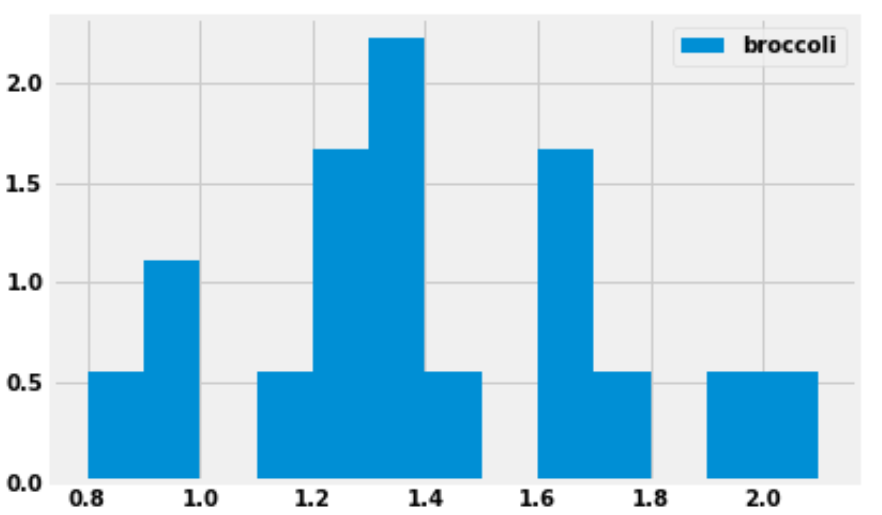How many grocery stores sold broccoli for a price greater than or equal to $1.30 per pound, but less than$1.40 per pound (the tallest bar)?

We are given that the bins start at 0.8 and have a width of 0.1, which means one of the bins has endpoints 1.3 and 1.4. This bin (the tallest bar) includes all grocery stores that sold broccoli for a price greater than or equal to $1.30 per pound, but less than$1.40 per pound.

This bar has a width of 0.1 and we’d estimate the height to be around 2.2, though we can’t say exactly. Multiplying these values, the area of the bar is about 0.22, which means about 22 percent of the grocery stores fall into this bin. There are 18 grocery stores in total, as we are told in the introduction to this question. We can compute using a calculator that 22 percent of 18 is 3.96. Since the actual number of grocery stores this represents must be a whole number, this bin must represent 4 grocery stores.

The reason for the slight discrepancy between 3.96 and 4 is that we used 2.2 for the height of the bar, a number that we determined by eye. We don’t know the exact height of the bar. It is reassuring to do the calculation and get a value that’s very close to an integer, since we know the final answer must be an integer.

##### Difficulty: ⭐️⭐️⭐️

The average score on this problem was 71%.

### Problem 5.2

Suppose we now plot the same data with different bins, using the following line of code:

prices.plot(kind='hist', y='broccoli', bins=[0.8, 1, 1.1, 1.5, 1.8, 1.9, 2.5], density=True)

What would be the height on the y-axis for the bin corresponding to the interval [\$1.10, \$1.50)? Input your answer below.

First, we need to figure out how many grocery stores the bin [\$1.10, \$1.50) contains. We already know from the previous subpart that there are four grocery stores in the bin [\$1.30, \$1.40). We could do similar calculations to find the number of grocery stores in each of these bins:

• [\$1.10, \$1.20)
• [\$1.20, \$1.30)
• [\$1.40, \$1.50)

However, it’s much simpler and faster to use the fact that when the bins are all equally wide, the height of a bar is proportional to the number of data values it contains. So looking at the histogram in the previous subpart, since we know the [\$1.30, \$1.40) bin contains 4 grocery stores, then the [\$1.10, \$1.20) bin must contain 1 grocery store, since it’s only a quarter as tall. Again, we’re taking advantage of the fact that there must be an integer number of grocery stores in each bin when we say it’s 1/4 as tall. Our only options are 1/4, 1/2, or 3/4 as tall, and among those choices, it’s clear.

Therefore, by looking at the relative heights of the bars, we can quickly determine the number of grocery stores in each bin:

• [\$1.10, \$1.20): 1 grocery store
• [\$1.20, \$1.30): 3 grocery stores
• [\$1.30, \$1.40): 4 grocery stores
• [\$1.40, \$1.50): 1 grocery store

Adding these numbers together, this means there are 9 grocery stores whose broccoli prices fall in the interval [\$1.10, \$1.50). In the new histogram, these 9 grocery stores will be represented by a bar of width 1.50-1.10 = 0.4. The area of the bar should be \frac{9}{18} = 0.5. Therefore the height must be \frac{0.5}{0.4} = 1.25.

##### Difficulty: ⭐️⭐️⭐️⭐️

The average score on this problem was 33%.

### Problem 5.3

You are interested in finding out the number of stores in which a pint of ice cream was cheaper than a pound of broccoli. Will you be able to determine the answer to this question by looking at the plot produced by the code below?

prices.get(['broccoli', 'ice_cream']).plot(kind='barh')
• Yes

• No

When we use .plot without specifying a y column, it uses every column in the DataFrame as a y column and creates an overlaid plot. Since we first use get with the list ['broccoli', 'ice_cream'], this keeps the 'broccoli' and 'ice_cream' columns from prices, so our bar chart will overlay broccoli prices with ice cream prices. Notice that this get is unnecessary because prices only has these two columns, so it would have been the same to just use prices directly. The resulting bar chart will look something like this: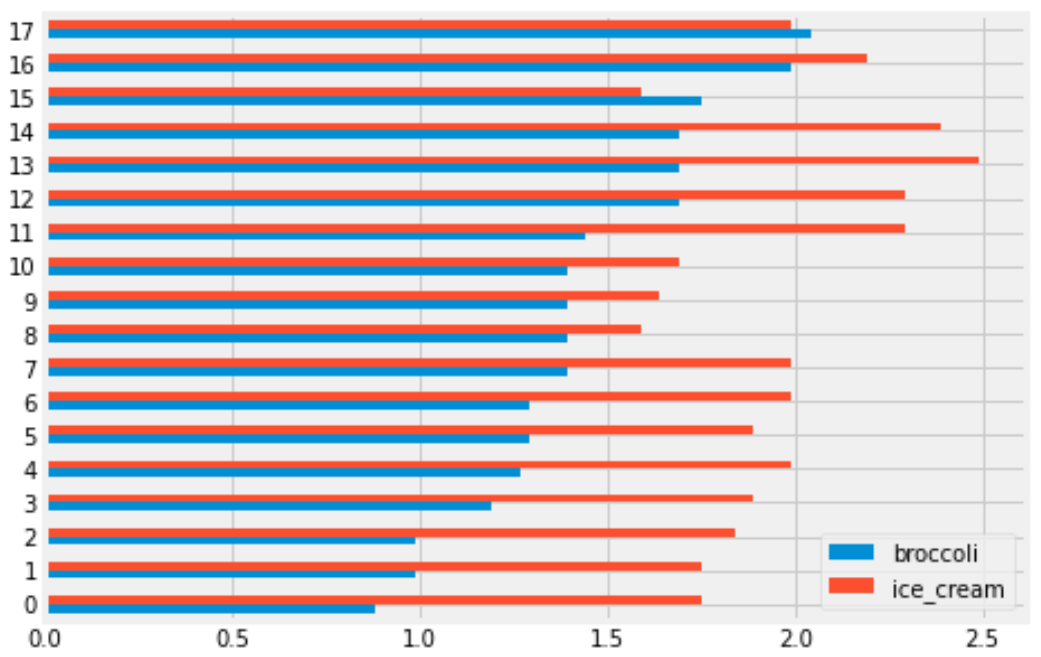Each grocery store has its broccoli price represented by the length of the blue bar and its ice cream price represented by the length of the red bar. We can therefore answer the question by simply counting the number of red bars that are shorter than their corresponding blue bars.

##### Difficulty: ⭐️⭐️

The average score on this problem was 78%.

### Problem 5.4

You are interested in finding out the number of stores in which a pint of ice cream was cheaper than a pound of broccoli. Will you be able to determine the answer to this question by looking at the plot produced by the code below?

prices.get(['broccoli', 'ice_cream']).plot(kind='hist')
• Yes

• No

This will create an overlaid histogram of broccoli prices and ice cream prices. So we will be able to see the distribution of broccoli prices together with the distribution of ice cream prices, but we won’t be able to pair up particular broccoli prices with ice cream prices at the same store. This means we won’t be able to answer the question. The overlaid histogram would look something like this: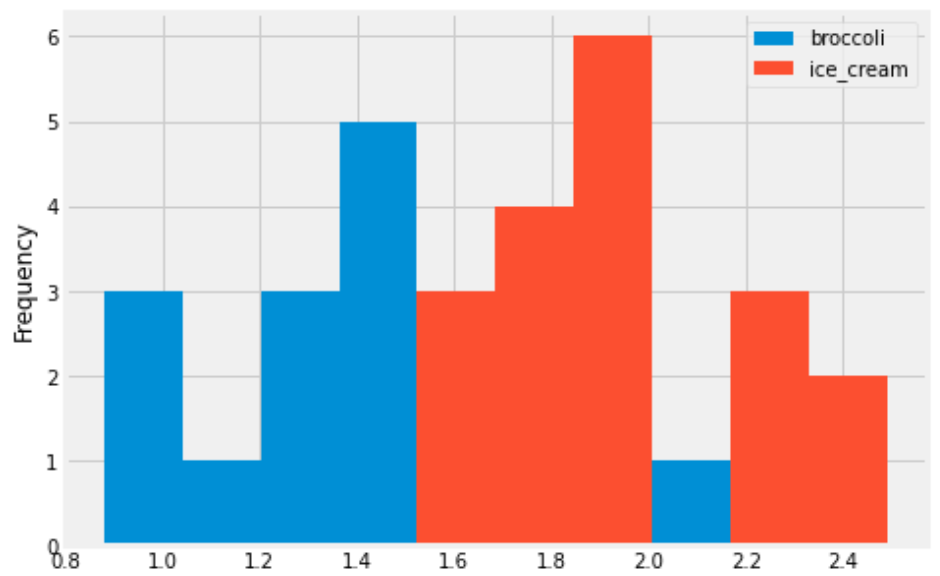This tells us that broadly, ice cream tends to be more expensive than broccoli, but we can’t say anything about the number of stores where ice cream is cheaper than broccoli.

##### Difficulty: ⭐️⭐️

The average score on this problem was 81%.

### Problem 5.5

Some code and the scatterplot that produced it is shown below:

(prices.get(['broccoli', 'ice_cream']).plot(kind='scatter', x='broccoli', y='ice_cream'))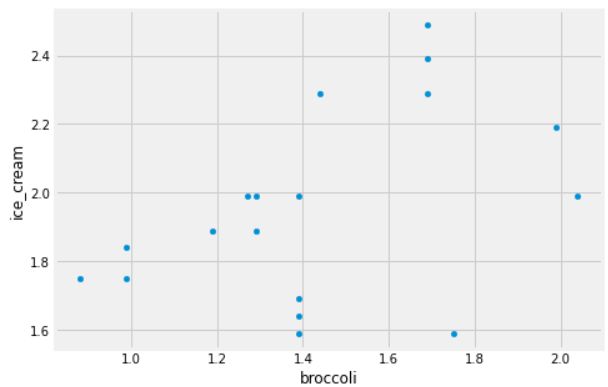Can you use this plot to figure out the number of stores in which a pint of ice cream was cheaper than a pound of broccoli?

If so, say how many such stores there are and explain how you came to that conclusion.

If not, explain why this scatterplot cannot be used to answer the question.

Answer: Yes, and there are 2 such stores.

In this scatterplot, each grocery store is represented as one dot. The x-coordinate of that dot tells the price of broccoli at that store, and the y-coordinate tells the price of ice cream. If a grocery store’s ice cream price is cheaper than its broccoli price, the dot in the scatterplot will have y<x. To identify such dots in the scatterplot, imagine drawing the line y=x. Any dot below this line corresponds to a point with y<x, which is a grocery store where ice cream is cheaper than broccoli. As we can see, there are two such stores.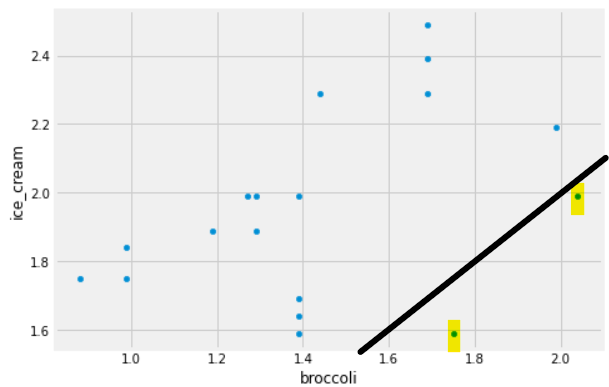##### Difficulty: ⭐️⭐️

The average score on this problem was 78%.

## Problem 6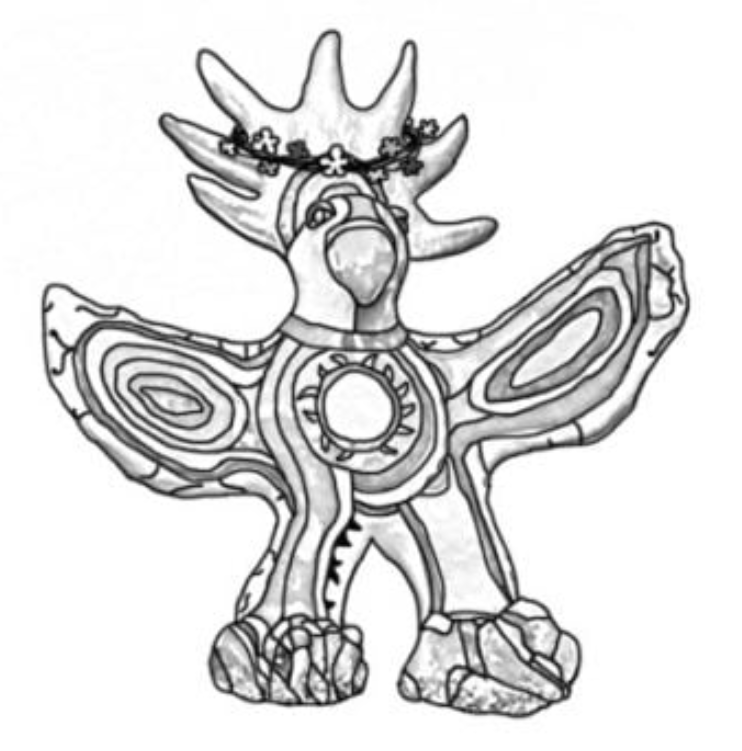Included is a DataFrame named sungod that contains information on the artists who have performed at Sun God in years past. For each year that the festival was held, we have one row for each artist that performed that year. The columns are:

• 'Year' (int): the year of the festival
• 'Artist' (str): the name of the artist
• 'Appearance_Order' (int): the order in which the artist appeared in that year’s festival (1 means they came onstage first)

The rows of sungod are arranged in no particular order. The first few rows of sungod are shown below (though sungod has many more rows than pictured here).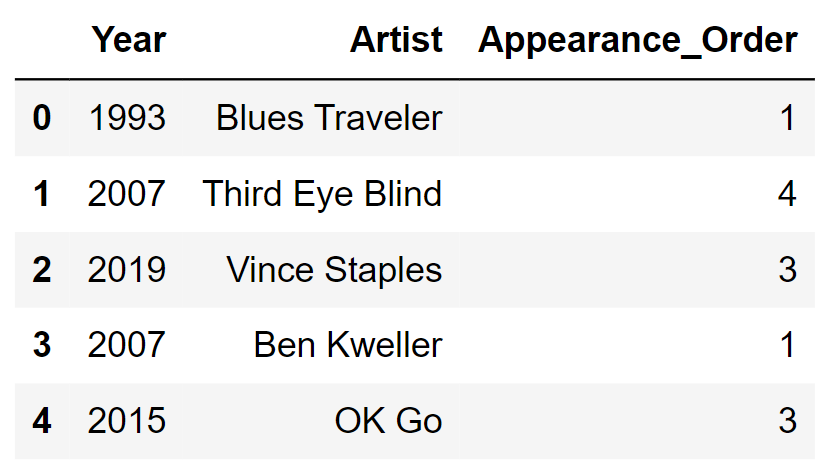Assume:

• Only one artist ever appeared at a time (for example, we can’t have two separate artists with a 'Year' of 2015 and an 'Appearance_Order' of 3).

• An artist may appear in multiple different Sun God festivals (they could be invited back).

• We have already run import babypandas as bpd and import numpy as np.

On the graph paper below, draw the histogram that would be produced by this code.

(
sungod.take(np.arange(5))
.plot(kind='hist', density=True,
bins=np.arange(0, 7, 2), y='Appearance_Order');
)

In your drawing, make sure to label the height of each bar in the histogram on the vertical axis. You can scale the axes however you like, and the two axes don’t need to be on the same scale.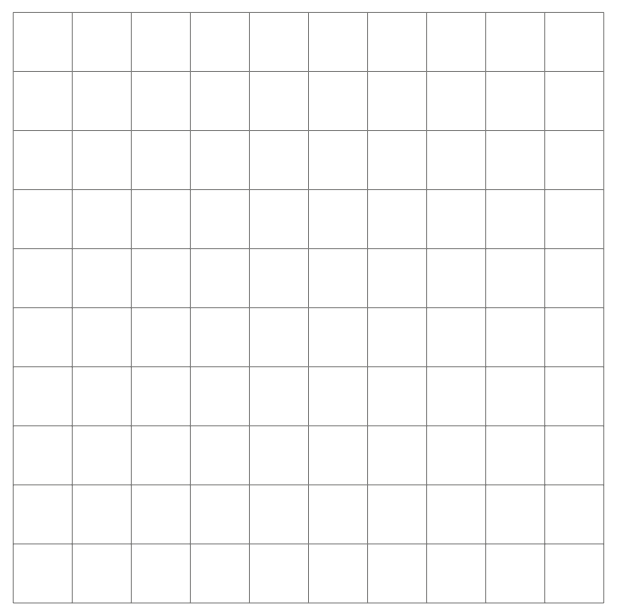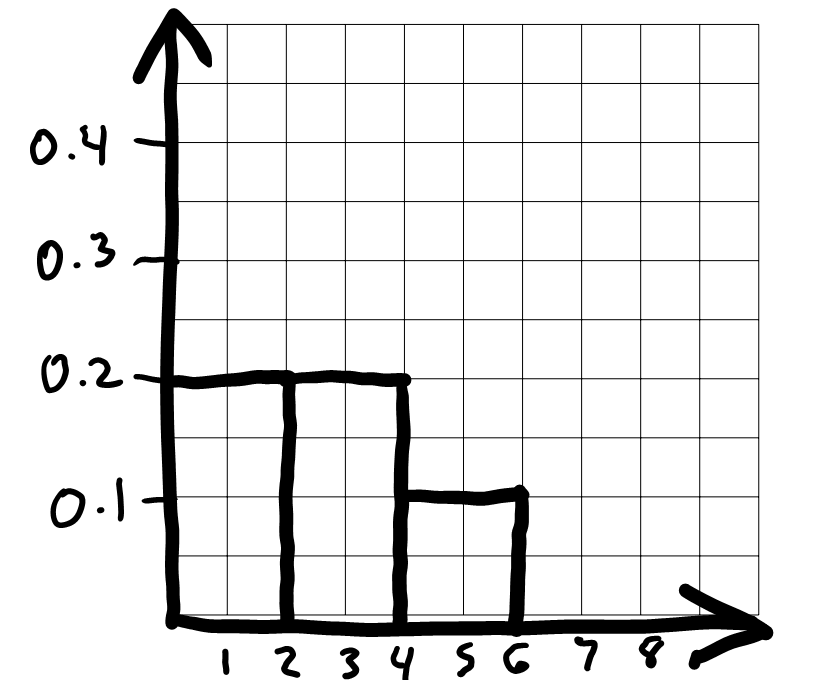To draw the histogram, we first need to bin the data and figure out how many data values fall into each bin. The code includes bins=np.arange(0, 7, 2) which means the bin endpoints are 0, 2, 4, 6. This gives us three bins: [0, 2), [2, 4), and [4, 6]. Remember that all bins, except for the last one, include the left endpoint but not the right. The last bin includes both endpoints.

Now that we know what the bins are, we can count up the number of values in each bin. We need to look at the 'Appearance_Order' column of sungod.take(np.arange(5)), or the first five rows of sungod. The values there are 1, 4, 3, 1, 3. The two 1s fall into the first bin [0, 2). The two 3s fall into the second bin [2, 4), and the one 4 falls into the last bin [4, 6]. This means the proportion of values in each bin are \frac{2}{5}, \frac{2}{5}, \frac{1}{5} from left to right.

To figure out the height of each bar in the histogram, we use the fact that the area of a bar in a density histogram should equal the proportion of values in that bin. The area of a rectangle is height times width, so height is area divided by width.

For the bin [0, 2), the area is \frac{2}{5} = 0.4 and the width is 2, so the height is \frac{0.4}{2} = 0.2.

For the bin [2, 4), the area is \frac{2}{5} = 0.4 and the width is 2, so the height is \frac{0.4}{2} = 0.2.

For the bin [4, 6], the area is \frac{1}{5} = 0.2 and the width is 2, so the height is \frac{0.2}{2} = 0.1.

Since the bins are all the same width, the fact that there an equal number of values in the first two bins and half as many in the third bin means the first two bars should be equally tall and the third should be half as tall. We can use this to draw the rest of the histogram quickly once we’ve drawn the first bar.

##### Difficulty: ⭐️⭐️⭐️⭐️

The average score on this problem was 45%.